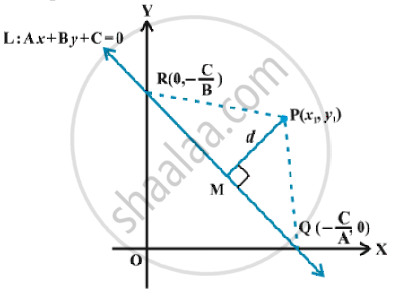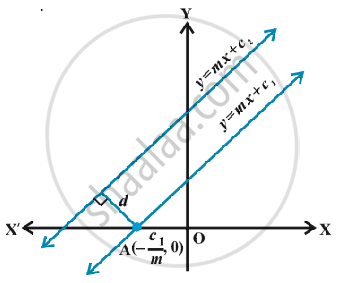# Distance of a Point from a Line

#### description

• Introduction of Distance of a Point from a Line
• Distance between two parallel lines

#### notes

Let L : Ax + By + C = 0 be a line, whose distance from the point P (x_1, y_1) is d. Draw a perpendicular PM from the point P to the line L in the Fig.the line meets the x-and y-axes at the points Q and R, respectively. Then, coordinates of the points are Q (-C/A,0) and R (0,-C/B). Thus the area of the triangle PQR is given by
area
(triangle PQR)=1/2PM .QR, which gives
PM=(2  area (triangle PQR))/(QR)  ...(1)
Also, area (triangle PQR) = 1/2 |x_1(0+C/B)+(-C/A)(-C/B-y_1)+0(y_1-0)|

=1/2|x_1C/B+y_1C/A +C_2/(AB)|

or 2area(trianglePQR) = |C/(AB)|.|Ax_1 + By_1+C|
and  QR = sqrt((0+C/A)^2 + (C/B-0)^2) = |C/(AB)| sqrt(A^2+B^2)
Substituting the values of area (∆PQR) and QR in (1), we get
PM = |Ax_1+By_1+C|/sqrt(A_2+B_2)
or

d=|Ax_1+By_1+C|/sqrt(A_2+B_2).
Thus, the Thus, the perpendicular distance (d) of a line Ax + By+ C = 0 from a point(x_1, y_1) is given by
d=|Ax_1+By_1+C|/sqrt(A_2+B_2)
Distance between two parallel lines :
The slopes of two parallel lines are equal. Therefore, two parallel lines can be taken in the form
y = mx+c_1    ...(1)and
y = mx +c_2   ...(2)
Line (1) will intersect x-axis at the point
A(-c_1/m,0) as shown in fig.Distance between two lines is equal to the length of the perpendicular from point A to line (2). Therefore, distance between the lines (1) and (2) is

|(-m)(-c_1/m)+(-c_2)|/sqrt(1+m_2) or d = |c_1-c_2|/sqrt(1+m_2)

Thus, the distance d between two parallel lines y= mx+ c_1 and   y= mx c_2 = + is given by

d = |c_1-c_2|sqrt(1+m_2).

If lines are given in general form, i.e.,  Ax + By + C_1 = 0 and Ax + By + C_2 = 0,
then above formula will take the form d = |c_1-C_2|/sqrt(A_2+B_2).

If you would like to contribute notes or other learning material, please submit them using the button below.

### Shaalaa.com

Distance of point from line [00:00:01]
S
0%